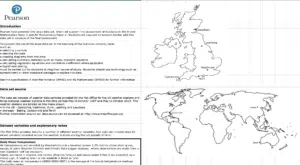# A-Level Maths (first teaching from 2017)

A-Level Maths is typically taught over two years – AS Maths is taught in the first year followed by A2 Maths in the second year. Pure Maths, Statistics and Mechanics are taught in both of the A-Level Maths years. The content of these years can be split into the topic areas seen below. Click on the links to find out more about the topics or see the content for individual A-Level Maths lessons. On each of these pages you should find notes on A-Level Maths, helpful examples, informative videos, questions by topic and much more.  To find out more about the A-Level Maths exams go to the AS Maths page or the A2 Maths page. Also see below for what you need to know on the Edexcel Large Dataset.

## Topics Covered in A2 Mechanics

1. A2 Maths Quantities & Units in Mechanics (not covered in A2 Maths)
2. A2 Maths KINEMATICS 2D kinematics
3. A2 Maths FORCES & NEWTON’S LAWS 2D forces
4. A2 Maths MOMENTS moments
5.

## The Large Dataset‘Big data’ is no longer a thing of the future and is creeping into A-Level Maths specifications across the board. You may be expected to answer questions based on a dataset that you are already familiar with. The more you are familiar with it, the more of an advantage you will have when answering questions. You may also be expected to recall trends in the data without being given the data.

The following links are provided by CrashMaths and provide guidance on the A-Level Maths Edexcel large dataset. This dataset contains weather statistics provided by the MET Office: